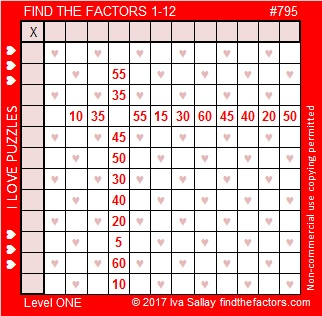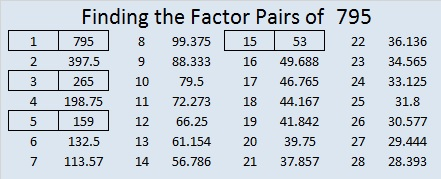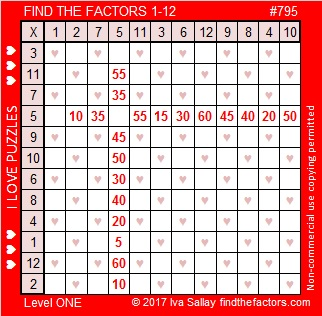# 795 a Level 1 Puzzle with ♥

I learned yesterday that pushing ALT on the computer key pad and 3 on the number pad at the same time produces a when the keys are released. I added a few ‘s to today’s puzzle because Valentine’s day is almost here:• 795 is a composite number.
• Prime factorization: 795 = 3 x 5 x 53
• The exponents in the prime factorization are 1, 1, and 1. Adding one to each and multiplying we get (1 + 1)(1 + 1)(1 + 1) = 2 x 2 x 2 = 8. Therefore 795 has exactly 8 factors.
• Factors of 795: 1, 3, 5, 15, 53, 159, 265, 795
• Factor pairs: 795 = 1 x 795, 3 x 265, 5 x 159, or 15 x 53
• 795 has no square factors that allow its square root to be simplified. √795 ≈ 28.195744.OEIS.org informs us that if we add up all the factors of 795 we get a number that is a perfect 4th power:

• 1 + 3 + 5 + 15 + 53 + 159 + 265 + 795 = 1296, which happens to be the 4th power of 6. (Of course 1296 also equals 36 squared.)

795 is the hypotenuse of four Pythagorean triples and thus:

• 69 – 792 – 795 which is 3 times (23 – 264 – 265)
• 288 – 741 – 795 which is 3 times (96 – 247 – 265)
• 420 – 675 – 795 which is 15 times (28 – 45 – 53)
• 477 – 636 – 795 which is 159 times (3 – 4 – 5)

795 is also the sum of three squares two different ways:

• 25² + 13² + 1² = 795
• 25² + 11² + 7² = 795## 2 thoughts on “795 a Level 1 Puzzle with ♥”

1.Steve Morris

I tried this, but it only seems to work if I use the number pad on the right hand side of the keyboard ♥

•ivasallay

Oh! Thanks for letting me know! I probably use the number pad at the right more than the keys at the top of the keyboard to type numbers. Without your comment, I may have never caught that only the number pad will produce a ♥.

This site uses Akismet to reduce spam. Learn how your comment data is processed.